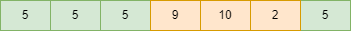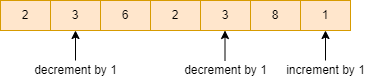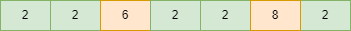# Maximize the frequency of an element by at most one increment or decrement of all array elements

## Introduction

### Problem statement

We are given an array. Our task is to maximize the frequency of any element in the given array by incrementing or decrementing each element by 1 at most one time.

### Sample examples

Input1:  a[] = [ 6, 5, 4, 9, 10, 2, 5]

Output1: 4

Explanation:Modified array:Maximum frequency = 4

Input2: a[] = [ 2, 3, 6, 2, 3, 8, 1]

Output2:  5

Explanation:Modified array:## Approach

Let’s suppose element “x” is the most frequent element in the given array after incrementing or decrementing each element by 1 at most one time. Now, if any element with the value “x-1” exists in the array, we increment it by 1 to make it “x”.

Similarly, if any element with the value “x+1” exists in the array, we decrement it by 1 to make it “x”.

To make element “x” the most frequent element, we need to consider the frequencies of all the numbers x-1, x and x+1.

Now, the maximum frequency after incrementing or decrementing each element by 1 at most one time is the sum of frequencies of x, x-1 and x+1.

Maximum frequency = frequency(“x”) + frequency(“x-1”) + frequency(“x+1”)

We can observe that x-1, x and x+1 are three consecutive numbers. So, we will use this crucial observation to solve the problem.

### Steps of Algorithm

• Find the minimum and maximum value in the given array and store it in minm and maxm variables.
• Declare a freq array of size = (maxm-minm+1) to store the frequency of each element, freq[maxm-minm+1] = {0}.
• Run a for loop and calculate the frequency of each element present in the array by incrementing freq[ a[i] - minm ] by 1 for every element.
• Calculate the sum of frequencies of all the pairs of three consecutive elements and find the maximum of these.

### Implementation in C++

``````#include<bits/stdc++.h>
using namespace std;

int maxm_frequency(int a[], int n)
{
int maxm_freq = 0;
int maxm = INT_MIN;

int minm = INT_MAX;

for (int i = 0; i < n; i++)
{
maxm = max(maxm, a[i]);
minm = min(minm, a[i]);
}

int freq[maxm - minm + 1] = {0};

for (int i = 0; i < n; i++)
{
freq[a[i] - minm]++;
}

for (int i = 0; i < (maxm - minm - 1); i++)
{
int curr_max = freq[i] + freq[i + 1] + freq[i - 1];
maxm_freq = max(maxm_freq, curr_max);
}

return maxm_freq;
}

int main()
{
int a[] = { 2, 3, 6, 2, 3, 8, 1};  //given array

int n = sizeof(a) / sizeof(a);    // size of array

cout << maxm_frequency(a, n);

return 0;
}``````

Output:

``5``

#### Complexity Analysis

Time complexity: We are using two loops of size n and (maxm-minm-1), so the time complexity is O(max(n,|maxm-minm|)) where n is the number of elements, minm is the minimum value, and maxm is the maximum value in the given array.

Space complexity: O(|maxm-minm|), as we are using an array of size (maxm-minm-1) to store the frequencies of all elements.

Q1.How do you find the maximum frequency of an element in an array?

Ans:

• Calculate the frequency of each element in the given array.
• Store it in an array with an index number equal to the element
• Traverse the array and find the maximum value in the array.

Q2. How do you locate the K elements that occur most frequently?

Ans:

• To store key-value pairs, such as element-frequency pairs, create a Hashmap hm.
• From beginning to end, traverse the array.
• Update hm[array[i]]++ for each element in the array.
• Sort the vector in decreasing order of frequency after storing the element-frequency pair in a vector.

Q3.What are the basic operations supported by an array?

Ans:

• Traverse - it prints each element of the array one by one.
• Insertion - Inserts a new element at the specified index.
• Delete - Deletes an element at the specified index.
• Update - Updates an element at the specified index.
• Search - Searches for an element based on the given index or value.

## Key Takeaways

This article discussed the approach to maximize the frequency of an element by at most one increment or decrement of all array elements by an important observation with its C++ code.

If you are a beginner, interested in coding and want to learn DSA, you can look for our guided path for DSA, which is free!